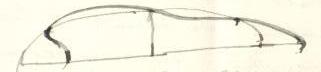# Transcription

## 3-15-13. George Howard Darwin to H. Poincaré

June 6. 1901

Newnham Grange — Cambridge

Dear Monsieur Poincaré,

I have been computing the change of moment of inertia in the pear. If $x$ is the axis of rotation the moment of inertia of the layer involves $y^{2}+z^{2}$, and thus is expressible as an harmonic of the second order.11Darwin 1902, 315 employs such an approach. Accordingly if the layer is concentrated in surface density on the ellipsoid, its m. of i. is zero, since the harmonic expressive of the layer is of the third order (of course you know this, but think it well to explain myself). The next approximation is found by concentrating this layer on a surface halfway between the ellipsoid and the true surface. That is to say I distort the layer by half the normal displacement.

Now I think one can see by general considerations that this distortion must give rise to $+$ m. of i. The distortion throughout all the long part of the ellipsoid must necessarily be almost without effect in creating m. of i. because the motion of the matter (whether positive or negative) leaves its distance from the axis of $x$ very nearly unchanged. But the conditions are different at the ends. At the sharp end we have positive matter moved further from $x$; and at the blunt end negative matter moved nearer to $x$. Both conspire to generate $+$ moment of inertia (and this I take it disposes of Schwarzschild).22See the figure in the notes to (§ 3-15-11), where the $x$-axis corresponds to the axis labelled $OA$. In the figure shown here, the $x$-axis is vertical; cf. the horizontal axis of the figure in Darwin to Poincaré, 12.08.1901 (§ 3-15-23). Darwin will note later (Darwin 1903, 313) that distortion is greatest at the extremities. For Darwin, an increase in moment of inertia implies the stability of the pear-shaped figure, and moots thereby the objection raised by Schwarzschild (cf. § 3-15-11). The rigorous formula for the moment of inertia computed in the way I have pointed out, is of most tremendous complication when expressed (as I am able to do) in terms of elliptic integrals $E$, $F$.33Cf. Darwin (1902, 313), the elliptic integrals are $E=\int_{0}^{\gamma}\sqrt{1-\kappa^{2}sin^{2}\psi}d\psi\quad\text{and}\quad F=% \int_{0}^{\gamma}\frac{d\psi}{\sqrt{1-\kappa^{2}sin^{2}\psi}},$ where $\gamma$ and $\kappa$ are given by: $\kappa^{2}=\frac{1-\beta}{1+\beta};\qquad\nu_{0}=\frac{1}{\kappa\sin\gamma},$ where $\beta$ is determined by the axes of the ellipsoid of reference (cf. the introduction), and $\nu_{0}$ is the parameter of the critical Jacobian (Darwin 1902, 320). On Darwin’s use of elliptic integrals, see (§ 3-15-43). But I should be sorry for any one who undertook to compute from this formula. I have however reduced this result to the determinn of certain integrals by quadratures which are not very laborious and I have today done the work once and obtain the positive result foreseen. I do not rely on the exact number until the whole is reworked.

There is one thing that rather puzzles me. It seems to me that at the birth of the new figure the angular velocity is stationary; I will not trouble you with my argument but I should much like to know whether you think so too. If it is so, then the numerical value of my moment of inertia (varying as the square of the thickness of the layer) will enable me to say what is the thickness of the layer corresponding to given increment of moment of momentum. In any case the result will only be applicable for a small thickness of layer.

 The position of the nodal lines in the sketch I sent you is so near the ends of the ellipsoid that we are unable even to obtain a general idea of future changes in the figure by drawing the case of a very thick layer, for if we draw such an exaggerated figure we find that the blunt end will acquire a hollow or dimple in the middle thus:which is absurd.44On Darwin’s nodal lines, see the chapter introduction. Darwin did not reproduce these lines in the figure accompanying his article (reproduced in the notes to Darwin to Poincaré, 28.05.1901, § 3-15-11). Darwin later echoes this passage as follows: In this first approximation the positions of the nodal lines […] lie so near the ends that it is impossible to construct an exaggerated figure, for if we do so the blunt end acquires a dimple, which is absurd. (Darwin 1902, 328) Karl Schwarzschild found the presence of dimples to be a sign of instability: Die Bemerkung, dass eine verhältnismässig geringe Deformation bereits ein Grübchen am stumpfen Ende der Birne erzeugt, legt die Vermutung nahe, dass in der Reihe der Birnfiguren, selbst wenn die ersten stabil sind, die Stabilität nicht lange anhalten wird. Wenigstens kann man, wenn ich richtig voraussehe, beweisen, dass eine Figur mit Grübchen nicht stabil sein kann. Oder sollten schon so bald andere ellipsoidal harmonics ausser $\mathfrak{P}_{3}\mathfrak{Q}_{3}$ in Betracht kommen, dass in Wirklichkeit das Grübchen gar nicht auftritt? (Schwarzschild to Darwin, 22.04.1902, § 3-48-6) See also Schwarzschild to Poincaré, 22.04.1902 (§ 3-41-1).

I have materials for obtaining any number more new figures of equilibrium – all unstable – but is it worth while? You may imagine that the work is heavy.

Yours very sincerely,

G. H. Darwin

ALS 4p. Collection particulière, Paris 75017.

## References

• G. H. Darwin (1902) On the pear-shaped figure of equilibrium of a rotating mass of liquid. Philosophical Transactions of the Royal Society A 198, pp. 301–331.
• G. H. Darwin (1903) The stability of the pear-shaped figure of equilibrium. Philosophical Transactions of the Royal Society A 200, pp. 251–314.

#### Titre (dcterms:title)

George Howard Darwin à Henri Poincaré, 06 juin 1901

#### Incipit (ahpo:incipit)

I have been computing the change of moment of inertia in the pear.

1901-06-06

#### Type (ahpo:documentType)

fr Lettre autographe signée

13

CD n° 113

#### Droits (ahpo:rightsHolder)

Archives Henri Poincaré

4

en

Gharnati Ph.D.